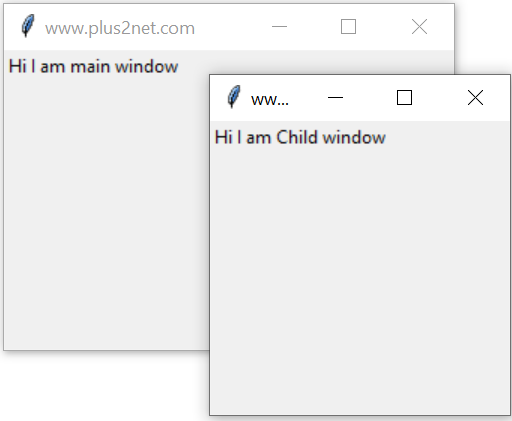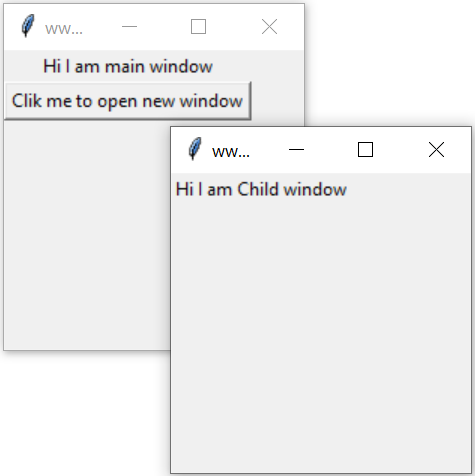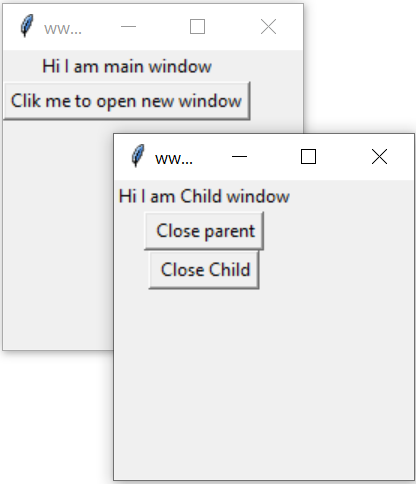# Python tkinter Toplevel for Child window

Tkinter
We can create pop-up window with all functionality by using Toplevel in Tkinter.Here is one sample code to create two windows from starting.
``````import tkinter as tk
#from tkinter import *

my_w = tk.Tk()
my_w.geometry("200x200")  # Size of the window

# create one lebel
my_str = tk.StringVar()
l1 = tk.Label(my_w,  textvariable=my_str )
l1.grid(row=1,column=2)
my_str.set("Hi I am main window")

# child window
my_w_child=Toplevel(my_w) # Child window
my_w_child.geometry("200x200")  # Size of the window
my_w_child.title("www.plus2net.com")

my_str1 = tk.StringVar()
l1 = tk.Label(my_w_child,  textvariable=my_str1 )
l1.grid(row=1,column=2)
my_str1.set("Hi I am Child window")

my_w.mainloop()``````
To manage layout read more on grid()

## Opening the Child window on Button clickAbove code will open both the windows ( parent and child ) at the beginning. We will change this by keeping the code for child window inside a function my_open(). We will use one button on parent window and click event of this will trigger the function my_open() to open the child window.
``````import tkinter as tk
from tkinter import *
my_w = tk.Tk()
my_w.geometry("200x200")  # Size of the window

# create one lebel
my_str = tk.StringVar()
l1 = tk.Label(my_w,  textvariable=my_str )
l1.grid(row=1,column=2)
my_str.set("Hi I am main window")
b1 = tk.Button(my_w, text='Clik me to open new window',
command=lambda:my_open())
b1.grid(row=2,column=2)

def my_open():
my_w_child=Toplevel(my_w) # Child window
my_w_child.geometry("200x200")  # Size of the window
my_w_child.title("www.plus2net.com")

my_str1 = tk.StringVar()
l1 = tk.Label(my_w_child,  textvariable=my_str1 )
l1.grid(row=1,column=2)
my_str1.set("Hi I am Child window")
my_w.mainloop()``````

## Child window with close buttonsOur child window can have two buttons, one button can close child window and other one can close parent window. While closing parent window child window will also close.
``````import tkinter as tk
from tkinter import *
my_w = tk.Tk()
my_w.geometry("200x200")  # Size of the window

# create one lebel
my_str = tk.StringVar()
l1 = tk.Label(my_w,  textvariable=my_str )
l1.grid(row=1,column=2)
my_str.set("Hi I am main window")
b1 = tk.Button(my_w, text='Clik me to open new window',
command=lambda:my_open())
b1.grid(row=2,column=2)

def my_open():
my_w_child=Toplevel(my_w) # Child window
my_w_child.geometry("200x200")  # Size of the window
my_w_child.title("www.plus2net.com")

my_str1 = tk.StringVar()
l1 = tk.Label(my_w_child,  textvariable=my_str1 )
l1.grid(row=1,column=2)
my_str1.set("Hi I am Child window")
b2 = tk.Button(my_w_child, text=' Close parent',
command=my_w.destroy)
b2.grid(row=2,column=2)

b3 = tk.Button(my_w_child, text=' Close Child',
command=my_w_child.destroy)
b3.grid(row=3,column=2)
my_w.mainloop()``````

## config

We can add options to our child window by using config. Here is a button to change the background colour of the child window.
``````b4 = tk.Button(my_w_child, text='Red',
command=lambda:my_w_child.config(bg='red'))``````
We can add one button from child on Parent. By controling the button at Parent we can change colour of Child window. Similarly by controling the button at Child we can change colour of Parent window.
``````    # change background color of parent from child
b4 = tk.Button(my_w_child, text='Parent Colour',
command=lambda:my_w.config(bg='red'))
b4.grid(row=4,column=2)
# change background color of child from parent
b5 = tk.Button(my_w, text='Child Colour',
command=lambda:my_w_child.config(bg='green'))
b5.grid(row=5,column=2)``````
Similarly other options are here

bg=Background color
bd=Border width
colormap=color map to use
container=container
cursor=style of mouse pointer
height=window height
highlightbackground=highlight the region when not in focus
highlightcolor=colour when in focusplus2net.com